Create a new printable

Black and white graphics
Color graphics

Select Currency
 US Dollar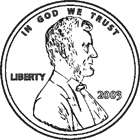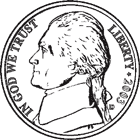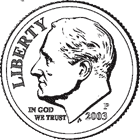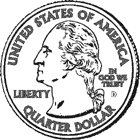Canadian Dollar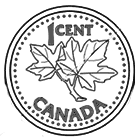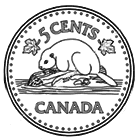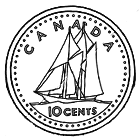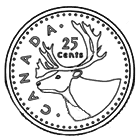Euro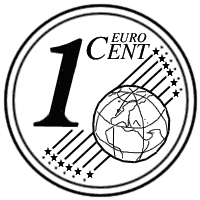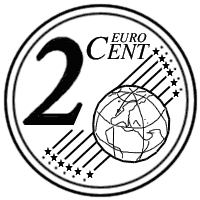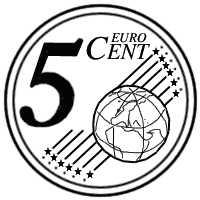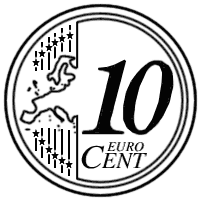Math Worksheets

Sample - Click above to make a new math worksheet (PDF).
 Name _____________________________Date ___________________
Dimes and Pennies
Fill in the missing value.

 1 * This is a pre-made sheet.Use the link at the top of the page for a printable page.
2.
 3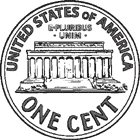+ _____= 43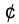3 pennies  + _____ dimes  = 433.
 9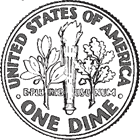+ 6= _____9 dimes  + 6 pennies  = _____4.
 _____+ 8= 82_____ pennies  + 8 dimes  = 825.
 7+ _____= 577 pennies  + _____ dimes  = 57Sample
This is only a sample worksheet.

Create a new printable
 US DollarCanadian DollarEuro